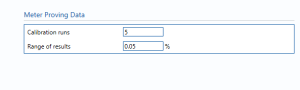# Coriolis Meter with Oil Prover

## Description

This block calculates the uncertainty in a Coriolis meter with an oil prover where mass flow rate uncertainties are added up to get the expanded uncertainty.

## Meter Proving Data### Calibration runs

Enter the number of runs that is made by the prover to calibrate the Coriolis meter.

### Range of results

Enter the percentage change in the range of results achieved.

## Uncertainty ParametersT/P corrections – The pressure and temperature corrections.

Drift – Allowance for drift. Normally left at zero, unless evidence is present to suggest a higher value.

Installation effects – Additional uncertainty due to the installation.

For example: piping configuration, stress, vibration.

Pulse count – The uncertainty in number of pulses recorded during proving.

Computation – Uncertainty due to computational errors in flow computer. Negligible term included for completeness – assume 0.01%.

## Calculations

The Liquid mass flow rates are calculated.

### Mass flow rate

The uncertainty calculation for the mass flow rate is detailed in the first uncertainty budget table giving a break down of how the overall uncertainty is calculatedThe values input into the uncertainty budget are taken from the uncertainty components calculated in this block from the calculation inputs. These values are taken in as the expanded uncertainties and are divided by a coverage factor to gain the standard uncertainty.

The coverage factor is determined by the probability distribution that best suits that uncertainty component. The standard uncertainty is then multiplied by the sensitivity value then squared. This is done for each component that contributes to the overall uncertainty in mass flow rate. The Standard Uncertainty in mass flow rate is the square root of the sum of each component variance as shown in the following equation:### Calculated UncertaintyThe Expanded Uncertainty is the Standard Uncertainty multiplied by the coverage factor (k). The coverage factor is defaulted to k = 2 (equivalent to a confidence level of approximately 95%).

The Relative Uncertainty is the Expanded Uncertainty divided by the mass flow rate.

### Standards

ISO 5168:2005 – Measurement of fluid flow – Procedures for the evaluation of uncertainties

ISO Guide 98-3 – Uncertainty of measurement – Part 3: Guide to the expression of uncertainty in measurement (GUM:1995)

Back to Uncertainty Modules